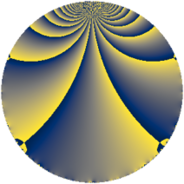# Properties

 Label 78.2.gLevel $78$ Weight $2$ Character orbit 78.g Rep. character $\chi_{78}(5,\cdot)$ Character field $\Q(\zeta_{4})$ Dimension $12$ Newform subspaces $1$ Sturm bound $28$ Trace bound $0$

# Related objects

## Defining parameters

 Level: $$N$$ $$=$$ $$78 = 2 \cdot 3 \cdot 13$$ Weight: $$k$$ $$=$$ $$2$$ Character orbit: $$[\chi]$$ $$=$$ 78.g (of order $$4$$ and degree $$2$$) Character conductor: $$\operatorname{cond}(\chi)$$ $$=$$ $$39$$ Character field: $$\Q(i)$$ Newform subspaces: $$1$$ Sturm bound: $$28$$ Trace bound: $$0$$

## Dimensions

The following table gives the dimensions of various subspaces of $$M_{2}(78, [\chi])$$.

Total New Old
Modular forms 36 12 24
Cusp forms 20 12 8
Eisenstein series 16 0 16

## Trace form

 $$12 q - 12 q^{7} + O(q^{10})$$ $$12 q - 12 q^{7} - 12 q^{16} - 12 q^{19} - 36 q^{27} + 12 q^{28} + 12 q^{31} + 36 q^{33} + 12 q^{37} + 36 q^{42} + 36 q^{45} + 12 q^{52} - 36 q^{54} - 36 q^{57} - 36 q^{63} - 12 q^{67} - 12 q^{73} - 12 q^{76} - 36 q^{78} + 72 q^{79} - 72 q^{85} - 12 q^{91} + 36 q^{93} - 72 q^{94} - 60 q^{97} + 36 q^{99} + O(q^{100})$$

## Decomposition of $$S_{2}^{\mathrm{new}}(78, [\chi])$$ into newform subspaces

Label Dim $A$ Field CM Traces $q$-expansion
$a_{2}$ $a_{3}$ $a_{5}$ $a_{7}$
78.2.g.a $12$ $0.623$ 12.0.$$\cdots$$.52 None $$0$$ $$0$$ $$0$$ $$-12$$ $$q+\beta _{2}q^{2}-\beta _{4}q^{3}+\beta _{8}q^{4}+(-\beta _{1}-\beta _{11})q^{5}+\cdots$$

## Decomposition of $$S_{2}^{\mathrm{old}}(78, [\chi])$$ into lower level spaces

$$S_{2}^{\mathrm{old}}(78, [\chi]) \cong$$ $$S_{2}^{\mathrm{new}}(39, [\chi])$$$$^{\oplus 2}$$